Reinforcement learning (RL) has been used successfully for solving tasks which have a well defined reward function – think AlphaZero for Go, OpenAI Five for Dota, or AlphaStar for StarCraft. However, in many practical situations you don’t have a well defined reward function. Even a task as seemingly straightforward as cleaning a room has many subtle cases: should a business card with a piece of gum be thrown away as trash, or might it have sentimental value? Should the clothes on the floor be washed, or returned to the closet? Where are notebooks supposed to be stored? Even when these aspects of a task have been clarified, translating it into a reward is non-trivial: if you provide rewards every time you sweep the trash, then the agent might dump the trash back out so that it can sweep it up again.1

Alternatively, we can try to learn a reward function from human feedback about the behavior of the agent. For example, Deep RL from Human Preferences learns a reward function from pairwise comparisons of video clips of the agent’s behavior. Unfortunately, however, this approach can be very costly: training a MuJoCo Cheetah to run forward requires a human to provide 750 comparisons.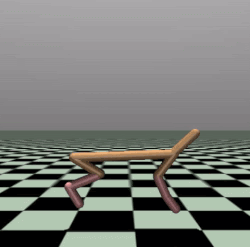Instead, we propose an algorithm that can learn a policy without any human supervision or reward function, by using information implicitly available in the state of the world. For example, we learn a policy that balances this Cheetah on its front leg from a single state in which it is balancing.

# Learning preferences by looking at the world

Humans seem to infer what other people want without needing much explicit supervision. How do we do it? As a concrete example, let’s imagine that you walk into a room, and you see this:You’re probably going to immediately be a lot more careful, to ensure you don’t knock down the elaborate house of cards.2 But how exactly did you know that you shouldn’t knock it down? Presumably you’ve never encountered a situation like this before, so it can’t be past experience. Nor can it be “built-in priors” from evolution – our hunter-gatherer ancestors did not routinely find giant houses of cards while foraging. No, the reason you know it should not be knocked down is that someone has clearly put in a lot of effort into making this house of cards – it certainly didn’t build itself – and they wouldn’t have done so unless they really cared about it.

Preferences Implicit in the State of the World develops an algorithm, Reward Learning by Simulating the Past (RLSP), that does this sort of reasoning, allowing an agent to infer human preferences without explicit feedback. As an example, consider the room environment below: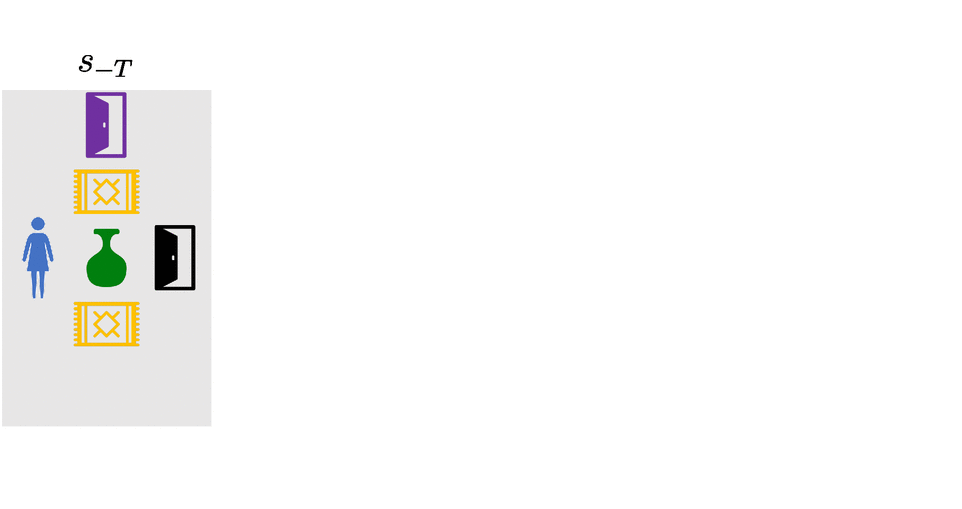When the robot is deployed, Alice asks it to navigate to the purple door. If we were to encode this as a reward function that only rewards the robot while it is at the purple door, the robot would take the shortest path to the purple door, knocking over and breaking the vase – since no one said it shouldn’t do that. The robot is perfectly aware that its plan causes it to break the vase, but by default it doesn’t realize that it shouldn’t break the vase.

RLSP can instead infer that the vase should not be broken. At a high level, it effectively considers all the ways that the past could have been, checks which ones are consistent with the observed state, and infers a reward function based on the result. If Alice didn’t care about whether the vase was broken, she would have probably broken it some time in the past. If she wanted the vase broken, she definitely would have broken it some time in the past. So the only consistent explanation is that Alice cared about the vase being intact. In contrast, we would observe the same state regardless of Alice’s preferences about carpets, and so RLSP does not infer anything about those preferences.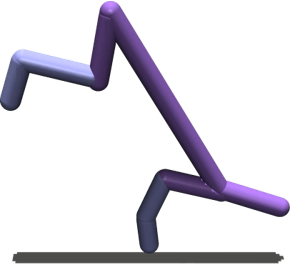Unfortunately, this approach requires reasoning about all possible pasts, which is intractable in even moderate environments. Prior work has only tested the idea in very simple gridworld environments. What would it take to scale this idea up to bigger, continuous environments, where we don’t have full knowledge of the environment dynamics? Intuitively, it should still be possible to make such inferences. Consider for example this Cheetah that is balancing on its front leg. Just as before, we can reason that there are very few behaviors that end up with the Cheetah in this particular state, and so the Cheetah “prefers” to be balancing on one leg.

In our latest paper presented at ICLR 2021, we introduce Deep Reward Learning by Simulating the Past (Deep RLSP), an extension of the RLSP algorithm that can be scaled up to tasks like the balancing Cheetah task.

# Simulating the past

The key difficulty for scaling up RLSP to bigger environments is in how to reason about “what must have happened in the past”. To address this, we sample likely past trajectories, instead of enumerating all possible past trajectories. In the case of the balancing Cheetah, we can infer that the Cheetah must have followed a trajectory similar to the one shown here: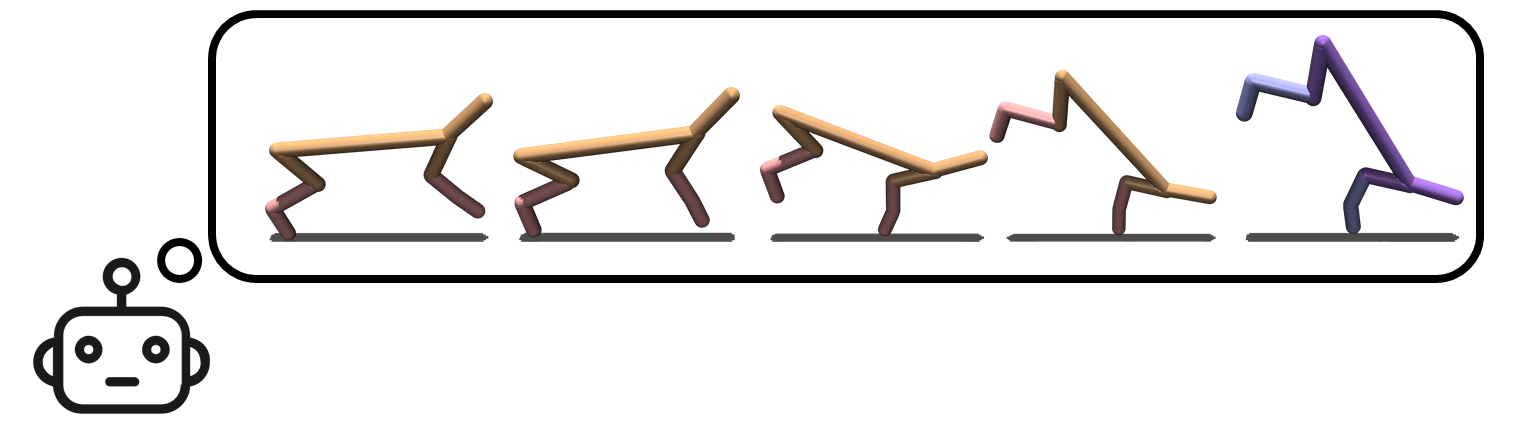Model-based RL algorithms often simulate the future by rolling out a policy $\pi(a_t \mid s_t)$ to choose actions and an environment dynamics model $\mathcal{T}(s_{t+1} \mid s_t, a_t)$ to predict future states. Similarly, we simulate the past by rolling out an inverse policy $\pi^{-1}(a_t \mid s_{t+1})$ that predicts which action $a_t$ the user took that resulted in the state $s_{t+1}$, and an inverse environment dynamics model $\mathcal{T}^{-1}(s_t \mid s_{t+1}, a_t)$ that predicts the state $s_t$ from which the chosen action $a_t$ would have led to $s_{t+1}$. By alternating between predicting past actions, and predicting past states from which those actions were taken, we can simulate trajectories arbitrarily far into the past.

Before we get into the details of how we train these models, let’s first understand how we’re going to use the trained models to infer preferences from an observed state $s_0$.

# The Deep RLSP gradient estimator

The RLSP algorithm uses gradient ascent to continuously update a linear reward function to explain an observed state $s_0$. To scale this idea up we make two key changes to their approach: (1) we learn a feature representation of each state, and model the reward function as linear in these features, and (2) we approximate the RLSP gradient by sampling likely past trajectories instead of enumerating all possible past trajectories. See our paper for a detailed discussion of the derivation.

This results in the Deep RLSP gradient estimator that aims to maximize the likelihood of an observed state $s_0$ under a reward function defined with a parameter vector $\theta$:

$\displaystyle \nabla_{\theta} \ln p(s_0 \mid \theta) = {\color{Blue}\mathop{\mathbb{E}}\limits_{\tau_{-T:-1}~\sim~\langle s_0, \pi^{-1}, \mathcal{T}^{-1} \rangle}} \left[ {\color{ForestGreen}\left( \sum_{t=-T}^{0} \phi(s_t) \right)} - {\color{Brown}\mathop{\mathbb{E}}\limits_{\tau'~\sim~\langle s_{-T}, \pi, \mathcal{T} \rangle}} \left[ {\color{Red}\left( \sum_{t=-T}^{0} \phi(s'_t) \right)} \right] \right]$

Intuitively the gradient is computed in three steps: First, we simulate backwards to determine what must have happened before $s_0$. Second, we simulate forwards to determine what the current policy (which is optimized for $\theta$) does. Third, we compute the difference of the backward and forward trajectories. This gradient changes the reward parameter $\theta$ such that it rewards the features observed in the backward trajectories, and punishes the features observed in the forward trajectories. As a result, when the reward is reoptimized, the new policy will tend to create trajectories that are less like the forward trajectories and more like the backward trajectories.

In other words, the gradient encourages a reward function such that the backward trajectories (what must have been done in the past) and forward trajectories (what an agent would do using the current reward) are consistent with each other. Once the trajectories are consistent, the gradient becomes zero, and we have learned a reward function that is likely to cause the observed state $s_0$.

The core of our algorithm is to perform gradient ascent using this gradient. However, we need access to $\phi$, $\pi^{-1}$, $\mathcal{T}^{-1}$, $\pi$, and $\mathcal{T}$ to compute the gradient. We learn these models from an initial dataset $\mathcal{D}$ of environment interactions. Note that $\mathcal{D}$ need not involve any human input: in our experiments, we use rollouts of a random policy to produce $\mathcal{D}$. We can then learn the necessary models as follows:

1. The feature function $\phi$ can be trained by applying any self-supervised representation learning technique to $\mathcal{D}$. We use a Variational Autoencoder (VAE) in our experiments.
2. The forward policy $\pi$ is trained using deep RL. We use Soft-Actor-Critic (SAC) in our experiments.
3. The forward environment dynamics $\mathcal{T}$ do not need to be learned, as we have access to a simulator for the environment.
4. The inverse policy $\pi^{-1}$ is trained using supervised learning on $(s, a, s’)$ transitions collected when executing $\pi$.
5. The inverse environment dynamics $\mathcal{T}^{-1}$ is trained using supervised learning on $(s, a, s’)$ transitions in $\mathcal{D}$.

# Deep RLSP in MuJoCo

To test our algorithm, we applied it to tasks in the MuJoCo simulator. These environments are commonly used to benchmark RL algorithms, and a typical task would be to make simulated robots walk.

To evaluate Deep RLSP, we use RL to train policies that walk, run or hop forward, and then sample a single state from these policies. Deep RLSP must then use just that state to infer that it is supposed to make the simulated robot walk forward. Note that this task is a little easier than it sounds because the state information in MuJoCo not only contains joint positions, but also velocities, so a single state also provides some information about how the robot is moving.

Our experiments show that this works reasonably well. We tested it primarily for a Cheetah robot and a Hopper robot, and in both cases it did in fact learn to move forward. Of course, the learned policies don’t perform worse as well as policies that are directly trained on the true reward function.

But the whole point of Deep RLSP is to learn in situations where we don’t have a reward. So, as a more interesting test case, we used Deep RLSP to imitate behaviors from a single state that are hard to explicitly specify in a reward function. We generated a set of “skills” using an unsupervised skill discovery algorithm called DADS, including the balancing skill that we saw earlier. Again, we sampled a single state or a small number of states, and checked whether Deep RLSP would learn to mimic the skill.

Since we don’t have access to a true reward function for “balancing”, we do not have an obvious way to quantitatively evaluate the performance of Deep RLSP. We instead looked at videos of the learned policies and judged them qualitatively. For example, here is the original balancing Cheetah, alongside the behavior learned by Deep RLSP using a single input state: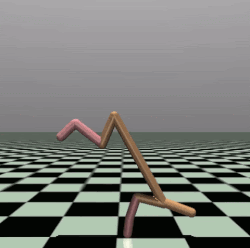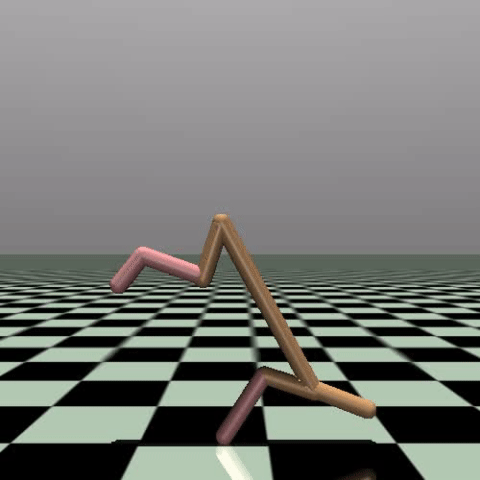From left to right: Original policy, Sampled state, and Deep RLSP policy.

The behavior isn’t perfect – you can see that the head sometimes touches the ground, and it doesn’t seem particularly good at balancing – but it has clearly learned the broad outline of what should be done.

# Looking forward

While our initial evaluation of Deep RLSP is promising, there is much remaining work for learning preferences from the state of the world.

The main requirement for Deep RLSP to work well is to learn good models of the inverse environment dynamics and inverse policy, and a good feature function. In the MuJoCo environments we relied on simple representation learning for the feature function and supervised learning for the models. This approach is unlikely to work for much bigger environments or real-world robotics applications. However, we are optimistic that advances in model-based RL can directly be applied to this problem.

A second open question is how to learn preferences from the state of the world in a multiagent setting. Typically the state will be optimized by one or more humans, and we want a different robot to learn these preferences. Deep RLSP currently learns the human’s reward and policy, but ultimately we want to use that to inform the robot’s behavior. In our experiments, the “human” and “robot” were the same, and so we could directly use the inferred policy as our robot policy, but obviously this will not be the case in a realistic application.

Finally, while we focused on imitation learning in this project, Deep RLSP is also very promising for learning safety constraints, such as “don’t break the vase”. We hope that the idea of learning preferences from the state of the world will also be useful for applying RL in safety critical environments.

This post is based on the paper “Learning What To Do by Simulating the Past”, presented at ICLR 2021. You can see our trained policies on our website. We also provide code to reproduce our experiments here.

1. See timestamp 31:47 in the linked podcast. Transcript: ‘One of the examples that I give is my friend and collaborator, Tom Griffiths. When his daughter was really young, she had this toy brush and pan, and she swept up some stuff on the floor and put it in the trash. And he praised her, like “Oh, wow, good job. You swept that really well.” And the daughter was very proud. And then without missing a beat, she dumps the trash back out onto the floor in order to sweep it up a second time and get the same praise a second time.’

2. Or maybe you immediately want to knock it down, because you like to see the world burn. Still, the point is that you do know that it counts as “destruction”, rather than just being a random side effect that nobody cares about.